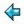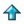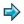### stack.m

```abstype stack *
with empty::stack *
push::*->stack *->stack *
pop::stack *->stack *
top::stack *->*
isempty::stack *->bool
showstack::(*->[char])->stack *->[char]

stack * == [*]
empty = []
push a x = a:x
pop(a:x) = x
top(a:x) = a
isempty x = (x=[])
showstack f [] = "empty"
showstack f (a:x) = "(push " ++ f a ++ " " ++ showstack f x ++ ")"

teststack = push 1 (push 2 (push 3 empty))
```

This script defines stack as an abstract data type, here based on lists. Note that there is a show function for stacks, causing them to print in a sensible way.